# Convolutional Neural Network

Some of the images and the content I used came from this great couple of blog posts  https://adeshpande3.github.io/adeshpande3.github.io/ and  the terrific book, "Neural Networks and Deep Learning" by Michael Nielsen. (Strongly recommend)

A convolutional neural network (CNN, or ConvNet) is a type of feed-forward artificial neural network in which the connectivity pattern between its neurons is inspired by the organization of the animal visual cortex.

The networks consist of multiple layers of small neuron collections which process portions of the input image, called receptive fields.

The outputs of these collections are then tiled so that their input regions overlap, to obtain a better representation of the original image; this is repeated for every such layer.

## How does it look like?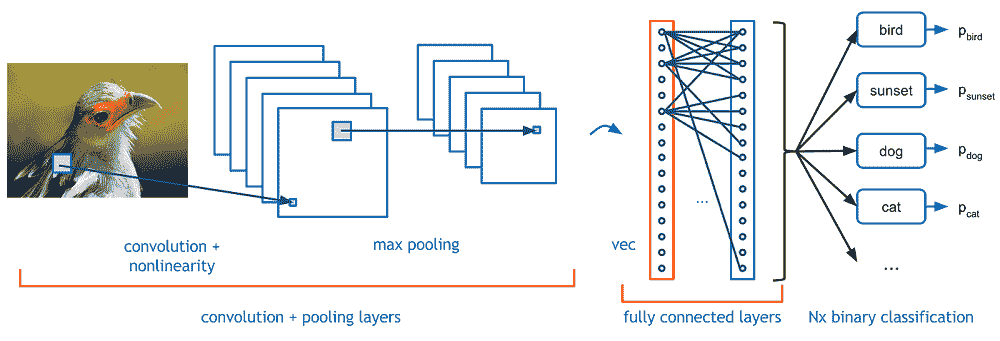# The Problem Space

## Image Classification

Image classification is the task of taking an input image and outputting a class (a cat, dog, etc) or a probability of classes that best describes the image.

For humans, this task of recognition is one of the first skills we learn from the moment we are born and is one that comes naturally and effortlessly as adults.

These skills of being able to quickly recognize patterns, generalize from prior knowledge, and adapt to different image environments are ones that we do not share with machines.

## Inputs and Outputs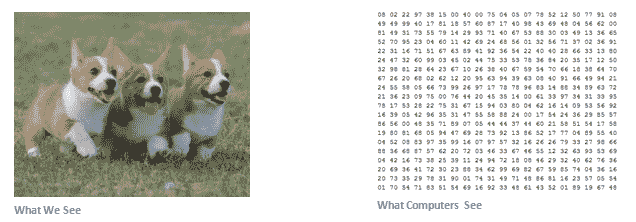When a computer sees an image (takes an image as input), it will see an array of pixel values.

Depending on the resolution and size of the image, it will see a 32 x 32 x 3 array of numbers (The 3 refers to RGB values).

let's say we have a color image in JPG form and its size is 480 x 480. The representative array will be 480 x 480 x 3. Each of these numbers is given a value from 0 to 255 which describes the pixel intensity at that point.

## Goal

What we want the computer to do is to be able to differentiate between all the images it’s given and figure out the unique features that make a dog a dog or that make a cat a cat.

When we look at a picture of a dog, we can classify it as such if the picture has identifiable features such as paws or 4 legs.

In a similar way, the computer should be able to perform image classification by looking for low level features such as edges and curves, and then building up to more abstract concepts through a series of convolutional layers.

## Structure of a CNN

A more detailed overview of what CNNs do would be that you take the image, pass it through a series of convolutional, nonlinear, pooling (downsampling), and fully connected layers, and get an output. As we said earlier, the output can be a single class or a probability of classes that best describes the image.

source: 

# Convolutional Layer

The first layer in a CNN is always a Convolutional Layer.### Convolutional filters

A Convolutional Filter much like a kernel in image recognition is a small matrix useful for blurring, sharpening, embossing, edge detection, and more.

This is accomplished by means of convolution between a kernel and an image.

#### The main difference here is that the conv matrices are learned.

As the filter is sliding, or convolving, around the input image, it is multiplying the values in the filter with the original pixel values of the image
(a.k.a. computing element wise multiplications).Now, we repeat this process for every location on the input volume. (Next step would be moving the filter to the right by 1 unit, then right again by 1, and so on).

After sliding the filter over all the locations, we are left with an array of numbers usually called an activation map or feature map.

## High Level Perspective

Let’s talk about briefly what this convolution is actually doing from a high level.

Each of these filters can be thought of as feature identifiers (e.g. straight edges, simple colors, curves)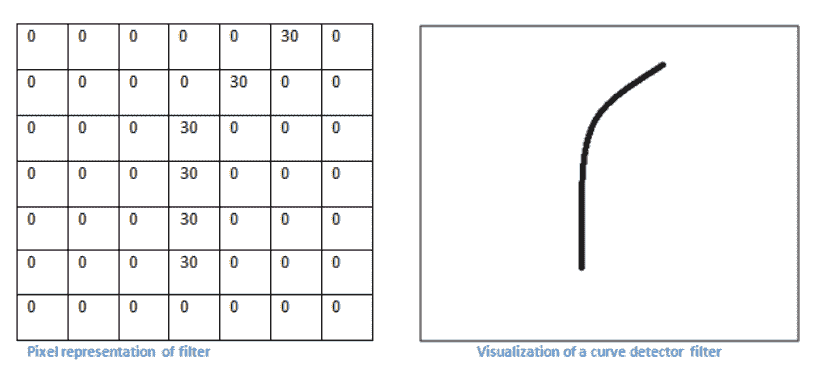### Visualisation of the Receptive Field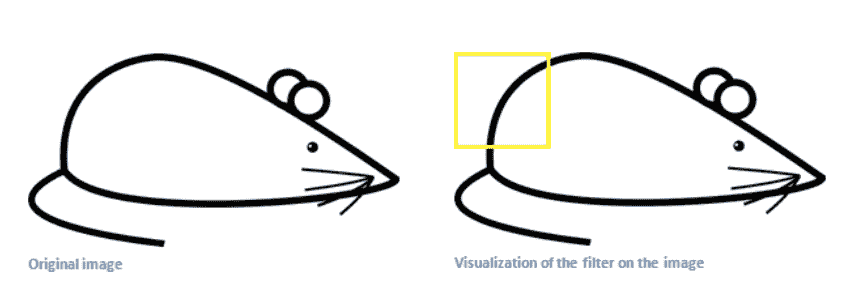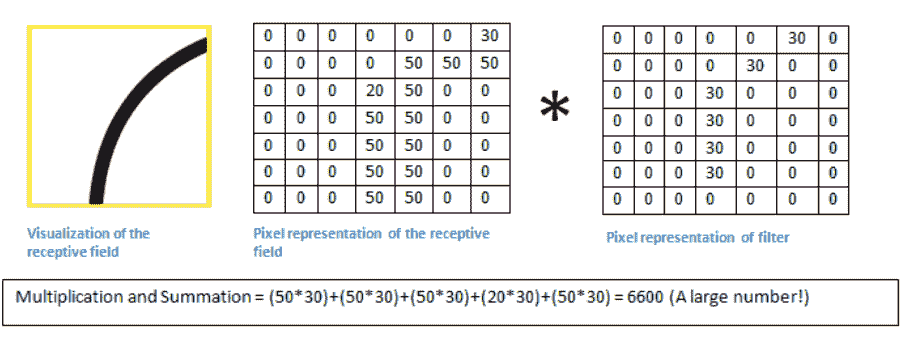The value is much lower! This is because there wasn’t anything in the image section that responded to the curve detector filter. Remember, the output of this conv layer is an activation map.

# Going Deeper Through the Network

Now in a traditional convolutional neural network architecture, there are other layers that are interspersed between these conv layers.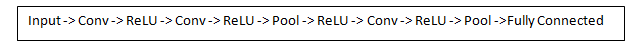## ReLU (Rectified Linear Units) Layer

After each conv layer, it is convention to apply a nonlinear layer (or activation layer) immediately afterward.

The purpose of this layer is to introduce nonlinearity to a system that basically has just been computing linear operations during the conv layers (just element wise multiplications and summations)

In the past, nonlinear functions like tanh and sigmoid were used, but researchers found out that ReLU layers work far better because the network is able to train a lot faster (because of the computational efficiency) without making a significant difference to the accuracy.

It also helps to alleviate the vanishing gradient problem, which is the issue where the lower layers of the network train very slowly because the gradient decreases exponentially through the layers

(very briefly)

Vanishing gradient problem depends on the choice of the activation function.

Many common activation functions (e.g sigmoid or tanh) squash their input into a very small output range in a very non-linear fashion.

For example, sigmoid maps the real number line onto a "small" range of [0, 1].

As a result, there are large regions of the input space which are mapped to an extremely small range.

In these regions of the input space, even a large change in the input will produce a small change in the output - hence the gradient is small.

### ReLu

The ReLu function is defined as $f(x) = \max(0, x),$ 

A smooth approximation to the rectifier is the analytic function: $f(x) = \ln(1 + e^x)$

which is called the softplus function.

The derivative of softplus is $f'(x) = e^x / (e^x + 1) = 1 / (1 + e^{-x})$, i.e. the logistic function.

 http://www.cs.toronto.edu/~fritz/absps/reluICML.pdf by G. E. Hinton

## Pooling Layers

After some ReLU layers, it is customary to apply a pooling layer (aka downsampling layer).

In this category, there are also several layer options, with maxpooling being the most popular.

Example of a MaxPooling filter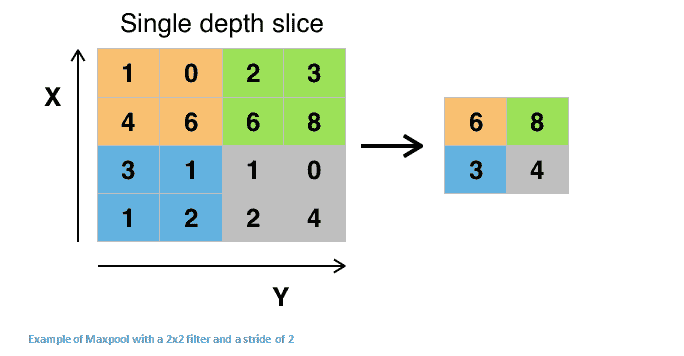Other options for pooling layers are average pooling and L2-norm pooling.

The intuition behind this Pooling layer is that once we know that a specific feature is in the original input volume (there will be a high activation value), its exact location is not as important as its relative location to the other features.

Therefore this layer drastically reduces the spatial dimension (the length and the width but not the depth) of the input volume.

This serves two main purposes: reduce the amount of parameters; controlling overfitting.

An intuitive explanation for the usefulness of pooling could be explained by an example:

Lets assume that we have a filter that is used for detecting faces. The exact pixel location of the face is less relevant then the fact that there is a face "somewhere at the top"

## Dropout Layer

The dropout layers have the very specific function to drop out a random set of activations in that layers by setting them to zero in the forward pass. Simple as that.

It allows to avoid overfitting but has to be used only at training time and not at test time.

## Fully Connected Layer

The last layer, however, is an important one, namely the Fully Connected Layer.

Basically, a FC layer looks at what high level features most strongly correlate to a particular class and has particular weights so that when you compute the products between the weights and the previous layer, you get the correct probabilities for the different classes.## Going further: Convolution Arithmetic

If you want to go further with Convolution and you want to fully understand how convolution works with all the details we omitted in this notebook, I strongly suggest to read this terrific paper: A guide to convolution arithmetic for deep learning.

This paper is also referenced (with animations) in the theano main documentation: convnet tutorial

# CNN in Keras

Keras has an extensive support for Convolutional Layers:

• 1D Convolutional Layers;
• 2D Convolutional Layers;
• 3D Convolutional Layers;
• Depthwise Convolution;
• Transpose Convolution;
• ....

The corresponding keras package is keras.layers.convolutional.

Take a look at the Convolutional Layers documentation to know more about Conv Layers that are missing in this notebook.

#### Convolution1D

from keras.layers.convolutional import Conv1D

dilation_rate=1, activation=None, use_bias=True,
kernel_initializer='glorot_uniform', bias_initializer='zeros',
kernel_regularizer=None, bias_regularizer=None,
activity_regularizer=None, kernel_constraint=None,
bias_constraint=None)


#### Arguments:

• filters: Integer, the dimensionality of the output space (i.e. the number output of filters in the convolution).
• kernel_size: An integer or tuple/list of a single integer, specifying the length of the 1D convolution window.
• strides: An integer or tuple/list of a single integer, specifying the stride length of the convolution. Specifying any stride value != 1 is incompatible with specifying any dilation_rate value != 1.
• padding: One of "valid", "causal" or "same" (case-insensitive). "causal" results in causal (dilated) convolutions, e.g. output[t] does not depend on input[t+1:]. Useful when modeling temporal data where the model should not violate the temporal order. See WaveNet: A Generative Model for Raw Audio, section 2.1.
• dilation_rate: an integer or tuple/list of a single integer, specifying the dilation rate to use for dilated convolution. Currently, specifying any dilation_rate value != 1 is incompatible with specifying any strides value != 1.
• activation: Activation function to use (see activations). If you don't specify anything, no activation is applied (ie. "linear" activation: a(x) = x).
• use_bias: Boolean, whether the layer uses a bias vector.
• kernel_initializer: Initializer for the kernel weights matrix (see initializers).
• bias_initializer: Initializer for the bias vector (see initializers).
• kernel_regularizer: Regularizer function applied to the kernel weights matrix (see regularizer).
• bias_regularizer: Regularizer function applied to the bias vector (see regularizer).
• activity_regularizer: Regularizer function applied to the output of the layer (its "activation"). (see regularizer).
• kernel_constraint: Constraint function applied to the kernel matrix (see constraints).
• bias_constraint: Constraint function applied to the bias vector (see constraints).

Convolution operator for filtering neighborhoods of one-dimensional inputs. When using this layer as the first layer in a model, either provide the keyword argument input_dim (int, e.g. 128 for sequences of 128-dimensional vectors), or input_shape (tuple of integers, e.g. (10, 128) for sequences of 10 vectors of 128-dimensional vectors).

#### Example


# apply a convolution 1d of length 3 to a sequence with 10 timesteps,
# with 64 output filters
model = Sequential()
# now model.output_shape == (None, 10, 64)

# add a new conv1d on top
# now model.output_shape == (None, 10, 32)


#### Convolution2D

from keras.layers.convolutional import Conv2D

data_format=None, dilation_rate=(1, 1), activation=None,
use_bias=True, kernel_initializer='glorot_uniform',
bias_initializer='zeros', kernel_regularizer=None,
bias_regularizer=None, activity_regularizer=None,
kernel_constraint=None, bias_constraint=None)


#### Arguments:

• filters: Integer, the dimensionality of the output space (i.e. the number output of filters in the convolution).
• kernel_size: An integer or tuple/list of 2 integers, specifying the width and height of the 2D convolution window. Can be a single integer to specify the same value for all spatial dimensions.
• strides: An integer or tuple/list of 2 integers, specifying the strides of the convolution along the width and height. Can be a single integer to specify the same value for all spatial dimensions. Specifying any stride value != 1 is incompatible with specifying any dilation_rate value != 1.
• padding: one of "valid" or "same" (case-insensitive).
• data_format: A string, one of channels_last (default) or channels_first. The ordering of the dimensions in the inputs. channels_last corresponds to inputs with shape (batch, height, width, channels) while channels_first corresponds to inputs with shape (batch, channels, height, width). It defaults to the image_data_format value found in your Keras config file at ~/.keras/keras.json. If you never set it, then it will be "channels_last".
• dilation_rate: an integer or tuple/list of 2 integers, specifying the dilation rate to use for dilated convolution. Can be a single integer to specify the same value for all spatial dimensions. Currently, specifying any dilation_rate value != 1 is incompatible with specifying any stride value != 1.
• activation: Activation function to use (see activations). If you don't specify anything, no activation is applied (ie. "linear" activation: a(x) = x).
• use_bias: Boolean, whether the layer uses a bias vector.
• kernel_initializer: Initializer for the kernel weights matrix (see initializers).
• bias_initializer: Initializer for the bias vector (see initializers).
• kernel_regularizer: Regularizer function applied to the kernel weights matrix (see regularizer).
• bias_regularizer: Regularizer function applied to the bias vector (see regularizer).
• activity_regularizer: Regularizer function applied to the output of the layer (its "activation"). (see regularizer).
• kernel_constraint: Constraint function applied to the kernel matrix (see constraints).
• bias_constraint: Constraint function applied to the bias vector (see constraints).

#### Example

Assuming keras.backend.image_data_format == "channels_last"


# apply a 3x3 convolution with 64 output filters on a 256x256 image:
model = Sequential()
input_shape=(3, 256, 256)))
# now model.output_shape == (None, 256, 256, 64)

# add a 3x3 convolution on top, with 32 output filters:
# now model.output_shape == (None, 256, 256, 32)


## Dimensions of Conv filters in Keras

The complex structure of ConvNets may lead to a representation that is challenging to understand.

Of course, the dimensions vary according to the dimension of the Convolutional filters (e.g. 1D, 2D)

### Convolution1D

Input Shape:

3D tensor with shape: (batch_size, steps, input_dim).

Output Shape:

3D tensor with shape: (batch_size, new_steps, filters).

### Convolution2D

Input Shape:

4D tensor with shape:

• (batch_size, channels, rows, cols) if image_data_format='channels_last'
• (batch_size, rows, cols, channels) if image_data_format='channels_first'

Output Shape:

4D tensor with shape:

• (batch_size, filters, new_rows, new_cols) if image_data_format='channels_first'
• (batch_size, new_rows, new_cols, filters) if image_data_format='channels_last'